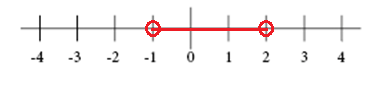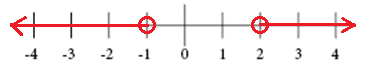# Solving compound inequalities

A compound inequality contains at least two inequalities that are separated by either "and" or "or".

The graph of a compound inequality with an "and" represents the intersection of the graph of the inequalities. A number is a solution to the compound inequality if the number is a solution to both inequalities. It can either be written as x > -1 and x < 2 or as -1 < x < 2.The graph of a compound inequality with an "or" represents the union of the graphs of the inequalities. A number is a solution to the compound inequality if the number is a solution to at least one of the inequalities. It is written as x < -1 or x > 2## Video lesson

Solve and graph the linear inequality

$-10 < 2 + x < -1$